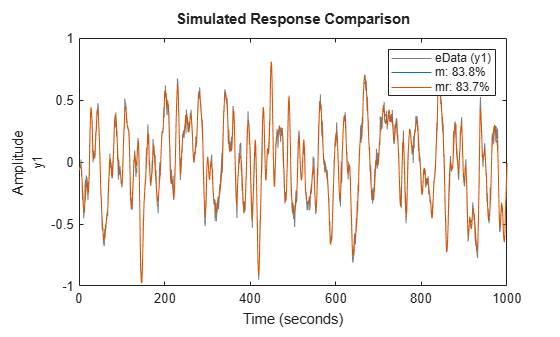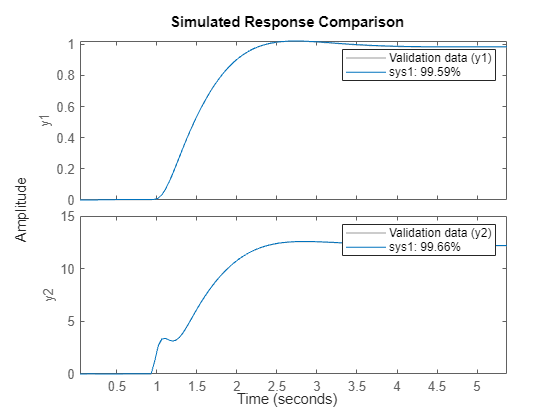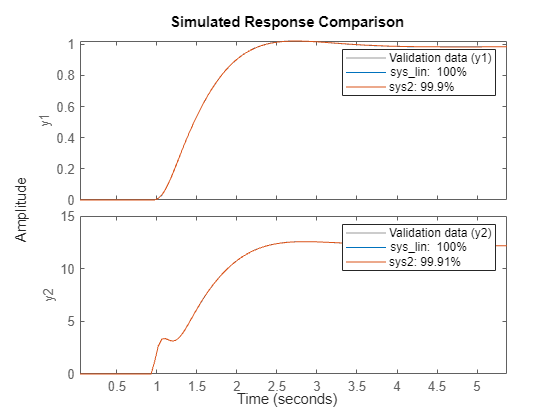Documentation

# ssest

Estimate state-space model using time or frequency domain data

## Syntax

``sys = ssest(data,nx)``
``sys = ssest(data,nx,Name,Value)``
``sys = ssest(___,opt)``
``sys = ssest(data,init_sys)``
``sys = ssest(data,init_sys,opt)``
``[sys,x0] = ssest(___)``

## Description

````sys = ssest(data,nx)` estimates a state-space model, `sys`, using time- or frequency-domain data, `data`. `sys` is a state-space model of order `nx` and represents:$\begin{array}{l}\stackrel{˙}{x}\left(t\right)=Ax\left(t\right)+Bu\left(t\right)+Ke\left(t\right)\\ y\left(t\right)=Cx\left(t\right)+Du\left(t\right)+e\left(t\right)\end{array}$A, B, C, D, and K are state-space matrices. u(t) is the input, y(t) is the output, e(t) is the disturbance and x(t) is the vector of `nx` states.All the entries of A, B, C, and K are free estimable parameters by default. D is fixed to zero by default, meaning that there is no feedthrough, except for static systems (`nx=0`).`sys = ssest(data,nx,Name,Value)` estimates the model using the additional options specified by one or more `Name,Value` pair arguments. Use the `Form`, `Feedthrough` and `DisturbanceModel` name-value pair arguments to modify the default behavior of the A, B, C, D, and K matrices.`sys = ssest(___,opt)` estimates the model using an option set, `opt`, that specifies options such as estimation objective, handling of initial conditions and numerical search method used for estimation.```
````sys = ssest(data,init_sys)` estimates a state-space model using the linear system `init_sys` to configure the initial parameterization.`sys = ssest(data,init_sys,opt)` estimates the model using an option set, `opt`.```
````[sys,x0] = ssest(___)` returns the value of initial states computed during estimation.```

## Input Arguments

collapse all

 `data` Estimation data. For time-domain estimation, `data` must be an `iddata` object containing the input and output signal values. For frequency-domain estimation, `data` can be one of the following: Recorded frequency response data (`frd` or `idfrd`)`iddata` object with its properties specified as follows:`InputData` — Fourier transform of the input signal`OutputData` — Fourier transform of the output signal`Domain` — `'Frequency'` `nx` Order of estimated model. Specify `nx` as a positive integer. `nx` may be a scalar or a vector. If `nx` is a vector, then `ssest` creates a plot which you can use to choose a suitable model order. The plot shows the Hankel singular values for models of different orders. States with relatively small Hankel singular values can be safely discarded. A default choice is suggested in the plot. `opt` Estimation options. `opt` is an options set, created using `ssestOptions`, that specifies options including: Estimation objectiveHandling of initial conditionsNumerical search method used for estimation If `opt` is not specified and `init_sys` is a previously estimated `idss` model, the options from `init_sys.Report.OptionsUsed` are used. `init_sys` Linear system that configures the initial parameterization of `sys`. You obtain `init_sys` by either performing an estimation using measured data or by direct construction. If `init_sys` is an `idss` model, `ssest` uses the parameter values of `init_sys` as the initial guess for estimating `sys`. For information on how to specify `idss`, see Estimate State-Space Models with Structured Parameterization. Constraints on the parameters of `init_sys`, such as fixed coefficients and minimum/maximum bounds are honored in estimating `sys`. Use the `Structure` property of `init_sys` to configure initial guesses and constraints for the A, B, C, D, and K matrices. For example: To specify an initial guess for the A matrix of `init_sys`, set `init_sys.Structure.A.Value` as the initial guess.To specify constraints for the B matrix of `init_sys`:Set `init_sys.Structure.B.Minimum` to the minimum B matrix valueSet `init_sys.Structure.B.Maximum` to the maximum B matrix valueSet `init_sys.Structure.B.Free` to indicate if entries of the B matrix are free parameters for estimation If `init_sys` is not a state-space (`idss`) model, the software first converts `init_sys` to an `idss` model. `ssest` uses the parameters of the resulting model as the initial guess for estimation. If `opt` is not specified, and `init_sys` was obtained by estimation, then the estimation options from `init_sys.Report.OptionsUsed` are used.

### Name-Value Pair Arguments

Specify optional comma-separated pairs of `Name,Value` arguments. `Name` is the argument name and `Value` is the corresponding value. `Name` must appear inside quotes. You can specify several name and value pair arguments in any order as `Name1,Value1,...,NameN,ValueN`.

Sample time, specified as a positive scalar.

For continuous-time models, use `Ts = 0`. For discrete-time models, specify `Ts` as a positive scalar whose value is equal to the data sample time.

Input delay for each input channel, specified as a numeric vector. For continuous-time systems, specify input delays in the time unit stored in the `TimeUnit` property. For discrete-time systems, specify input delays in integer multiples of the sample time `Ts`. For example, `InputDelay = 3` means a delay of three sampling periods.

For a system with `Nu` inputs, set `InputDelay` to an `Nu`-by-1 vector. Each entry of this vector is a numerical value that represents the input delay for the corresponding input channel.

You can also set `InputDelay` to a scalar value to apply the same delay to all channels.

Type of canonical form of `sys`, specified as one of the following values:

• `'modal'` — Obtain `sys` in modal form.

• `'companion'` — Obtain `sys` in companion form.

• `'free'` — All entries of the A, B and C matrices are treated as free.

• `'canonical'` — Obtain `sys` in the observability canonical form .

Use the `Form`, `Feedthrough` and `DisturbanceModel` name-value pair arguments to modify the default behavior of the A, B, C, D, and K matrices.

For more information, see Estimate State-Space Models with Canonical Parameterization.

Direct feedthrough from input to output, specified as a logical vector of length Nu, where Nu is the number of inputs. If `Feedthrough` is specified as a logical scalar, it is applied to all the inputs.

Use the `Form`, `Feedthrough` and `DisturbanceModel` name-value pair arguments to modify the default behavior of the A, B, C, D, and K matrices.

Specify whether to estimate the K matrix which specifies the noise component, specified as one of the following values:

• `'none'` — Noise component is not estimated. The value of the K matrix is fixed to zero value.

• `'estimate'` — The K matrix is treated as a free parameter.

`DisturbanceModel` must be `'none'` when using frequency-domain data.

Use the `Form`, `Feedthrough` and `DisturbanceModel` name-value pair arguments to modify the default behavior of the A, B, C, D, and K matrices.

## Output Arguments

`sys`

Identified state-space model, returned as a `idss` model. This model is created using the specified model orders, delays, and estimation options.

Information about the estimation results and options used is stored in the `Report` property of the model. `Report` has the following fields:

Report FieldDescription
`Status`

Summary of the model status, which indicates whether the model was created by construction or obtained by estimation.

`Method`

Estimation command used.

`InitialState`

How initial states were handled during estimation, returned as one of the following values:

• `'zero'` — The initial state is set to zero.

• `'estimate'` — The initial state is treated as an independent estimation parameter.

• `'backcast'` — The initial state is estimated using the best least squares fit.

• Column vector of length Nx, where Nx is the number of states. For multi-experiment data, a matrix with Ne columns, where Ne is the number of experiments.

• Parametric initial condition object (`x0obj`) created using `idpar`. Only for discrete-time state-space models.

This field is especially useful when the `InitialState` option in the estimation option set is `'auto'`.

`N4Weight`

Weighting scheme used for singular-value decomposition by the N4SID algorithm, returned as one of the following values:

• `'MOESP'` — Uses the MOESP algorithm.

• `'CVA'` — Uses the Canonical Variable Algorithm.

• `'SSARX'` — A subspace identification method that uses an ARX estimation based algorithm to compute the weighting.

This option is especially useful when the `N4Weight` option in the estimation option set is `'auto'`.

`N4Horizon`

Forward and backward prediction horizons used by the N4SID algorithm, returned as a row vector with three elements — ` [r sy su]`, where `r` is the maximum forward prediction horizon. `sy` is the number of past outputs, and `su` is the number of past inputs that are used for the predictions.

`Fit`

Quantitative assessment of the estimation, returned as a structure. See Loss Function and Model Quality Metrics for more information on these quality metrics. The structure has the following fields:

FieldDescription
`FitPercent`

Normalized root mean squared error (NRMSE) measure of how well the response of the model fits the estimation data, expressed as a percentage.

`LossFcn`

Value of the loss function when the estimation completes.

`MSE`

Mean squared error (MSE) measure of how well the response of the model fits the estimation data.

`FPE`

Final prediction error for the model.

`AIC`

Raw Akaike Information Criteria (AIC) measure of model quality.

`AICc`

Small sample-size corrected AIC.

`nAIC`

Normalized AIC.

`BIC`

Bayesian Information Criteria (BIC).

`Parameters`

Estimated values of model parameters.

`OptionsUsed`

Option set used for estimation. If no custom options were configured, this is a set of default options. See `ssestOptions` for more information.

`RandState`

State of the random number stream at the start of estimation. Empty, `[]`, if randomization was not used during estimation. For more information, see `rng` in the MATLAB® documentation.

`DataUsed`

Attributes of the data used for estimation. Structure with the following fields:

FieldDescription
`Name`

Name of the data set.

`Type`

Data type. For ARX models, this is set to ```'Time domain data'```.

`Length`

Number of data samples.

`Ts`

Sample time. This is equivalent to `Data.Ts`.

`InterSample`

Input intersample behavior. One of the following values:

• `'zoh'` — Zero-order hold maintains a piecewise-constant input signal between samples.

• `'foh'` — First-order hold maintains a piecewise-linear input signal between samples.

• `'bl'` — Band-limited behavior specifies that the continuous-time input signal has zero power above the Nyquist frequency.

The value of `Intersample` has no effect on estimation results for discrete-time models.

`InputOffset`

Offset removed from time-domain input data during estimation.

`OutputOffset`

Offset removed from time-domain output data during estimation.

`Termination`

Termination conditions for the iterative search used for prediction error minimization. Structure with the following fields:

FieldDescription
`WhyStop`

Reason for terminating the numerical search.

`Iterations`

Number of search iterations performed by the estimation algorithm.

`FirstOrderOptimality`

$\infty$-norm of the gradient search vector when the search algorithm terminates.

`FcnCount`

Number of times the objective function was called.

`UpdateNorm`

Norm of the gradient search vector in the last iteration. Omitted when the search method is `'lsqnonlin'` or `'fmincon'`.

`LastImprovement`

Criterion improvement in the last iteration, expressed as a percentage. Omitted when the search method is `'lsqnonlin'` or `'fmincon'`.

`Algorithm`

Algorithm used by `'lsqnonlin'` or `'fmincon'` search method. Omitted when other search methods are used.

For estimation methods that do not require numerical search optimization, the `Termination` field is omitted.

For more information on using `Report`, see Estimation Report.

`x0`

Initial states computed during the estimation.

If `data` contains multiple experiments, then `x0` is an array with each column corresponding to an experiment.

This value is also stored in the `Parameters` field of the model’s `Report` property.

## Examples

### Determine Optimal Estimated Model Order

Obtain measured input-output data.

```load icEngine.mat; data = iddata(y,u,0.04);```

`data` is an `iddata` object containing 1500 input-output data samples. The data sample time is 0.04 seconds.

Estimate a state-space model for measured input-output data. Determine the optimal model order within a given model order range.

```nx = 1:10; sys = ssest(data,nx);```

A plot that shows the Hankel singular values (SVD) for models of the orders specified by `nx` appears.States with relatively small Hankel singular values can be safely discarded. The suggested default order choice is `3`.

Select the model order in the Model Order drop-down list and click .

### Identify State-Space Model With Input Delay

Load time-domain system response data.

`load iddata7 z7;`

Identify a fourth-order state-space model of the data. Specify a known delay of `2` seconds for the first input and `0` seconds for the second input.

```nx = 4; sys = ssest(z7(1:300),nx,'InputDelay',[2;0]);```

### Estimate State-Space Model Using Regularization

Obtain a regularized 5th order state-space model for a 2nd order system from a narrow bandwidth signal.

Load estimation data.

`load regularizationExampleData eData;`

Create the transfer function model used for generating the estimation data (true system).

`trueSys = idtf([0.02008 0.04017 0.02008],[1 -1.561 0.6414],1);`

Estimate an unregularized state-space model.

```opt = ssestOptions('SearchMethod','lm'); m = ssest(eData,5,'form','modal','DisturbanceModel','none','Ts',eData.Ts,opt);```

Estimate a regularized state-space model.

```opt.Regularization.Lambda = 10; mr = ssest(eData,5,'form','modal','DisturbanceModel','none','Ts',eData.Ts,opt);```

Compare the model outputs with the estimation data.

`compare(eData,m,mr);`Compare the model impulse responses.

```impulse(trueSys,m,mr,50); legend('trueSys','m','mr');```### Estimate Partially Known State-Space Model Using Structured Estimation

Estimate a state-space model of measured input-output data. Configure the parameter constraints and initial values for estimation using a state-space model.

Create an `idss` model to specify the initial parameterization for estimation.

```A = blkdiag([-0.1 0.4; -0.4 -0.1],[-1 5; -5 -1]); B = [1; zeros(3,1)]; C = [1 1 1 1]; D = 0; K = zeros(4,1); x0 = [0.1 0.1 0.1 0.1]; Ts = 0; init_sys = idss(A,B,C,D,K,x0,Ts);```

Setting all entries of `K` to `0` creates an `idss` model with no state disturbance element.

Use the `Structure` property to fix the values of some of the model parameters. Configure the model so that `B` and `K` are fixed, and only the nonzero entries of `A` are estimable.

```init_sys.Structure.A.Free = (A~=0); init_sys.Structure.B.Free = false; init_sys.Structure.K.Free = false;```

The entries in `init_sys.Structure.A.Free` determine whether the corresponding entries in `init_sys.A` are free (`true`) or fixed (`false`).

Load the measured data and estimate a state-space model using the parameter constraints and initial values specified by `init_sys`.

```load iddata2 z2; sys = ssest(z2,init_sys);```

The estimated parameters of `sys` satisfy the constraints specified by `init_sys`.

### Model Order Reduction by Estimation

Consider the Simulink model `idF14Model`. Linearizing this model gives a ninth-order model. However, the dynamics of the model can be captured, without compromising the fit quality too much, using a lower-order model.

Obtain the linearized model.

```load_system('idF14Model'); io = getlinio('idF14Model'); sys_lin = linearize('idF14Model',io);```

`sys_lin` is a ninth-order state-space model with two outputs and one input.

Simulate the step response of the linearized model, and use the data to create an `iddata` object.

```Ts = 0.0444; t = (0:Ts:4.44)'; y = step(sys_lin,t); data = iddata([zeros(20,2);y],[zeros(20,1); ones(101,1)],Ts);```

`data` is an `iddata` object that encapsulates the step response of `sys_lin`.

Compare the data to the model linearization.

`compare(data,sys_lin);`Because the data was obtained by simulating the linearized model, there is a 100% match between the data and model linearization response.

Identify a state-space model with a reduced order that adequately fits the data.

Determine an optimal model order.

```nx = 1:9; sys1 = ssest(data,nx,'DisturbanceModel','none'); ```

A plot showing the Hankel singular values (SVD) for models of the orders specified by `nx` appears.States with relatively small Hankel singular values can be safely discarded. The plot suggests using a fifth-order model.

At the MATLAB command prompt, select the model order for the estimated state-space model. Specify the model order as `5`, or press Enter to use the default order value.

Compare the data to the estimated model.

`compare(data,sys1);``sys1` provides a 98.4% fit for the first output and a 97.7% fit for the second output.

Examine the stopping condition for the search algorithm.

`sys1.Report.Termination.WhyStop`
```ans = 'Maximum number of iterations reached.' ```

Create an estimation options set that specifies the `'lm'` search method and allows a maximum of 50 search iterations.

```opt = ssestOptions('SearchMethod','lm'); opt.SearchOptions.MaxIterations = 50; opt.Display = 'on';```

Identify a state-space model using the estimation option set and `sys1` as the estimation initialization model.

`sys2 = ssest(data,sys1,opt);`

Compare the response of the linearized and the estimated models.

`compare(data,sys_lin,sys2);``sys2` provides a 99% fit for the first output and a 98% fit for the second output while using 4 less states than `sys_lin` .

## More About

collapse all

### Modal Form

In modal form, A is a block-diagonal matrix. The block size is typically 1-by-1 for real eigenvalues and 2-by-2 for complex eigenvalues. However, if there are repeated eigenvalues or clusters of nearby eigenvalues, the block size can be larger.

For example, for a system with eigenvalues $\left({\lambda }_{1},\sigma ±j\omega ,{\lambda }_{2}\right)$, the modal A matrix is of the form

`$\left[\begin{array}{cccc}{\lambda }_{1}& 0& 0& 0\\ 0& \sigma & \omega & 0\\ 0& -\omega & \sigma & 0\\ 0& 0& 0& {\lambda }_{2}\end{array}\right]$`

### Companion Form

In the companion realization, the characteristic polynomial of the system appears explicitly in the right-most column of the A matrix.

For a system with characteristic polynomial

`$p\left(s\right)={s}^{n}+{\alpha }_{1}{s}^{n-1}+\dots +{\alpha }_{n-1}s+{\alpha }_{n}$`

the corresponding companion A matrix is

`$A=\left[\begin{array}{cccccc}0& 0& ..& ..& 0& -{\alpha }_{n}\\ 1& 0& 0& ..& 0& -{\alpha }_{n}-1\\ 0& 1& 0& .& :& :\\ :& 0& .& .& :& :\\ 0& .& .& 1& 0& -{\alpha }_{2}\\ 0& ..& ..& 0& 1& -{\alpha }_{1}\end{array}\right]$`

The companion transformation requires that the system be controllable from the first input. The companion form is poorly conditioned for most state-space computations; avoid using it when possible.

## Algorithms

`ssest` initializes the parameter estimates using either a noniterative subspace approach or an iterative rational function estimation approach. It then refines the parameter values using the prediction error minimization approach. See `pem` and `ssestOptions` for more information.

## References

 Ljung, L. System Identification: Theory For the User, Second Edition, Upper Saddle River, N.J: Prentice Hall, 1999.

## Extended Capabilities

#### Introduced in R2012a

##### SupportGet trial now